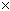SEARCH HOMEMath Central Quandaries & QueriesDear Sirs, Please could you help me with an arithmetic problem? I was told that if x > y (integers); then x would never exactly divide y^n (n integer > 1) if (x,y) = 1; or if x is "square free". Is the latter true and why? Many thanks, AndrewAndrew,

If (x,y) = 1 then no prime divisor of x appears in y and thus no prime divisor of x appears in yn so x cannot divide it - the x > y condition seems superfluous.

I'm not clear on what your second question is asking; are you saying that if x is squarefree then it doesn't divide yn and dropping the (x,y) = 1 condition? I think here you may want the x > y condition. If x was to divide yn and x was squarefree then what would that say about x dividing y? Now if x > y ... .

Penny

Andrew,

The Fundamental Theorem of Arithmetic (yes, that's its real name) says this:

Every natural number greater than 1 can be written as a unique product of prime numbers.

So it is true that if x > y > 1 and the lowest common factor of x and y is 1, then x does not divide y and therefore yn if n is an integer > 1. This is because the prime factors of yn are copies of the prime factors of y, none of which is shared by the prime factors of x.

[Your question has a twist to it however. You say x and y are integers, not natural numbers (whole numbers > 0). So does x = 1 and y = 0 work? Certainly x divides y, since 1 divides any integer evenly. Thus, x cannot equal one, so on this technicality, the statement is false.]

So can x divide yn if x > y when x, y, n are natural numbers > 1 and x is square free?

Let's say y = 6. Any value of n will give a prime factorization of yn = 2n 3n. What divides this? Only other numbers divisible by only 2s and/or 3s, due to the fundamental theorem of arithmetic.

So that means that any number x that divides yn can be written as 2i 3j where i and j are whole numbers. However, if i or j is larger than 1, then the number x is not square free. To keep it square free, i and j must less than 2. But that just means the only numbers possible are 1, 2, 3, or 23 which is 6. But that means x > y is violated. Thus, if x is square free, then it cannot divide yn.

So the latter part of the statement is true as a consequence of the Fundamental Theorem of Arithmetic as well as the first.

Hope this helps,
Stephen La Rocque.Math Central is supported by the University of Regina and The Pacific Institute for the Mathematical Sciences.